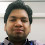1. Image Noise: The high frequency component of the image is called as noise. In other words noise can be defined as the abrupt changes in the intensity values of the pixel of image. This random variation in the image is called as noise.
2. Noise has bad effect on the image.
3. It degrades the quality of the image due to which it becomes difficult to interpret the image.

There are two types of noise models:
1. Additive noise is the undesired abrupt signal that gets added into some genuine signal.
2. The additive noise model is described as:
Noisy_image[t] = original_image[t] + noise[t]
where, original_image[t] is an unknown but deterministic image signal which is corrupted by a zero-mean noise process noise[t], and  is the observed, i.e., noisy signal. The main objective of the additive noise image restoration model is to restore the image signal original_image[t] based on the observations Noisy_image[t].
3. Removing additive noise from the image is not a very difficult task because there are many image restoration models available for eliminating additive noise.
4. Gaussian noise is best example of additive noise. This noise is present in many applications like optical imagery and few types of medical images like CT scan etc.
5. Main sources: during image acquisition.
6. The affect of this noise on image is less adverse than multiplicative noise as here the noisy signal gets added to original signal while in multiplicative, the noise is multiplied.
7. It has normal distribution pattern.

b) Multiplicative noise model:
1. Multiplicative noise is the undesired abrupt signal that gets multiplied into some genuine signal.
2. The multiplicative noise model is described as:
Noisy_image[t] = original_image[t] * noise[t]
where, original_image[t] is an unknown but deterministic image signal which is corrupted by a noise process i.e. noise[t], and  is the observed, i.e., noisy signal. The main objective of the multiplicative noise image restoration model is to restore the image signal original_image[t] based on the observations Noisy_image[t].
3. Removing multiplicative noise from the image is a very difficult task because there are very few image restoration models available to eliminate this kind of noise. Therefore working on this noise is much more challenging and good for new researchers because they have much bigger scope to work on.
4. There is one another way to handle this noise using image restoration models of additive noise. This way is achieved by converting the multiplicative nature into additive using logarithm transformation. Using log transform, the multiplicative noise converts to additive noise and now any filtering method can be applied to it. And later inverse log is used to get the correct result.
5. Main sources: during capture, transmission, or other processing.
6. This noise has adverse affect on the image as noisy signal gets multiplied to original signal.
7. It has gamma distribution pattern.
8. Speckle noise is the best example of multiplicative noise.
9. This kind of noise is mainly found in radar images and ultrasound images.
10. Research papers (eliminating multiplicative noise):
What are additive and multiplicative noise?Reviewed by IPR on April 16, 2020 Rating: 5

1.great...very useful information for my research work..Thanks so much..!!
Kindly post more..!!

2.Very helpful for my Research project
Thanks sir

3.Thanks for this information.Its very useful for my research work.